# Managerial Economics Business Strategy Chapter 11 Pricing Strategies

• Slides: 44Managerial Economics & Business Strategy Chapter 11 Pricing Strategies for Firms with Market Power Mc. Graw-Hill/Irwin Michael R. Baye, Managerial Economics and Business Strategy Copyright © 2008 by the Mc. Graw-Hill Companies, Inc. All rights reserved.11 -2 Overview I. Basic Pricing Strategies Monopoly & Monopolistic Competition Cournot Oligopoly II. Extracting Consumer Surplus Price Discrimination Block Pricing Two-Part Pricing Commodity Bundling III. Pricing for Special Cost and Demand Structures Peak-Load Pricing Cross Subsidies Transfer Pricing IV. Pricing in Markets with Intense Price Competition Price Matching Brand Loyalty Randomized Pricing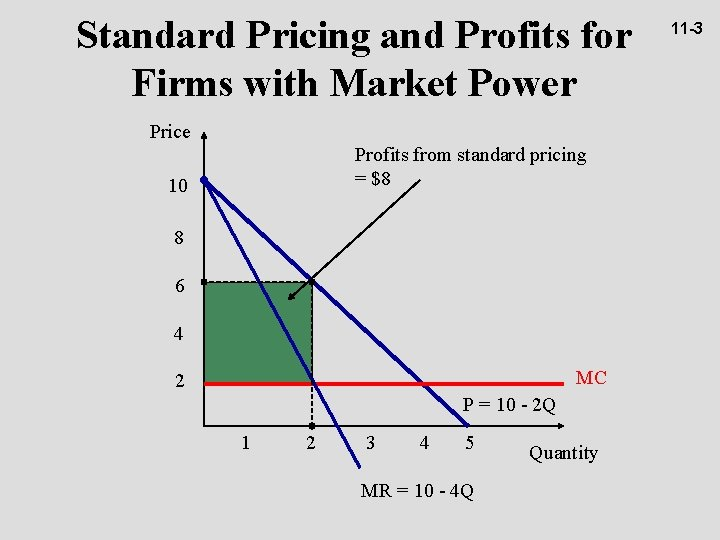Standard Pricing and Profits for Firms with Market Power Price Profits from standard pricing = \$8 10 8 6 4 MC 2 P = 10 - 2 Q 1 2 3 4 5 MR = 10 - 4 Q Quantity 11 -3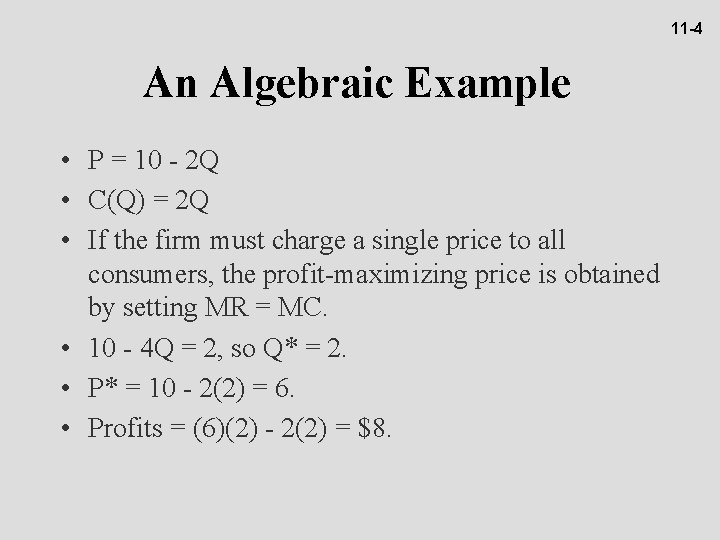11 -4 An Algebraic Example • P = 10 - 2 Q • C(Q) = 2 Q • If the firm must charge a single price to all consumers, the profit-maximizing price is obtained by setting MR = MC. • 10 - 4 Q = 2, so Q* = 2. • P* = 10 - 2(2) = 6. • Profits = (6)(2) - 2(2) = \$8.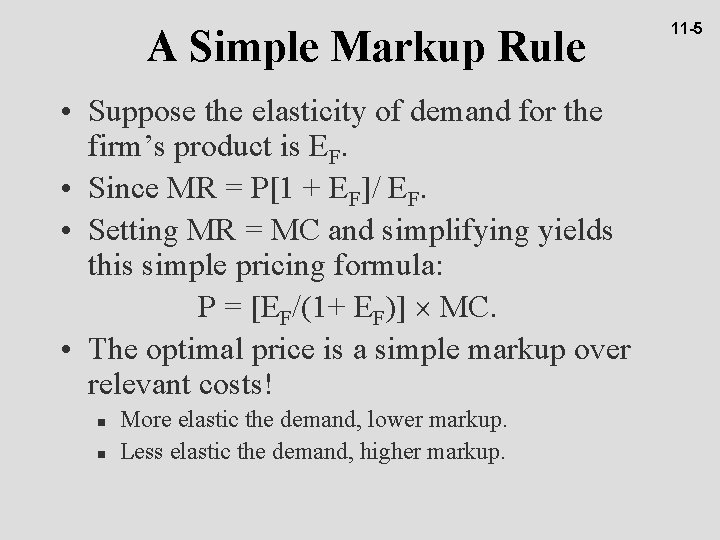A Simple Markup Rule • Suppose the elasticity of demand for the firm’s product is EF. • Since MR = P[1 + EF]/ EF. • Setting MR = MC and simplifying yields this simple pricing formula: P = [EF/(1+ EF)] MC. • The optimal price is a simple markup over relevant costs! More elastic the demand, lower markup. Less elastic the demand, higher markup. 11 -5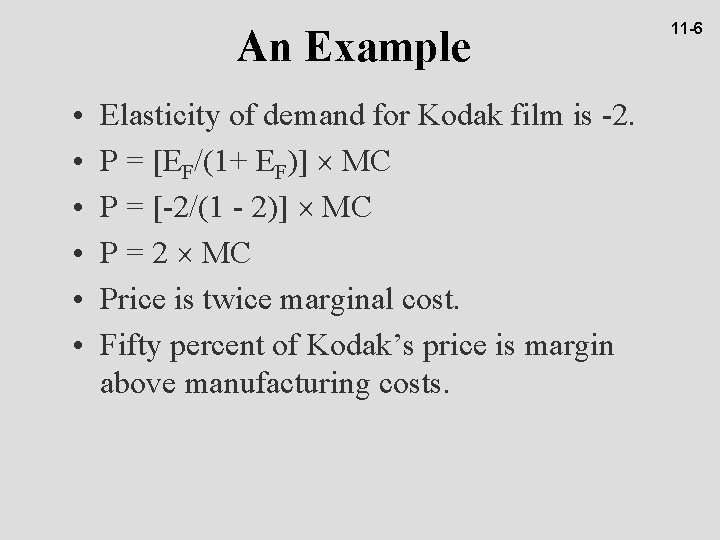An Example • • • Elasticity of demand for Kodak film is -2. P = [EF/(1+ EF)] MC P = [-2/(1 - 2)] MC P = 2 MC Price is twice marginal cost. Fifty percent of Kodak’s price is margin above manufacturing costs. 11 -6Markup Rule for Cournot Oligopoly • • Homogeneous product Cournot oligopoly. N = total number of firms in the industry. Market elasticity of demand EM. Elasticity of individual firm’s demand is given by EF = N x EM. • Since P = [EF/(1+ EF)] MC, • Then, P = [NEM/(1+ NEM)] MC. • The greater the number of firms, the lower the profit-maximizing markup factor. 11 -7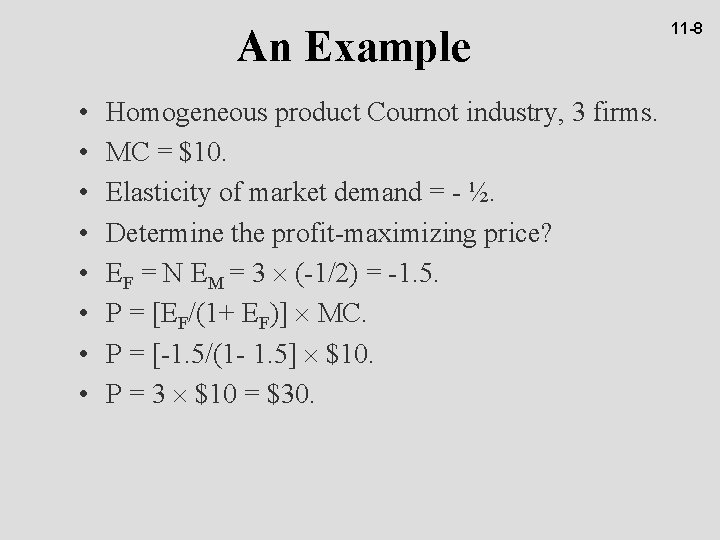An Example • • Homogeneous product Cournot industry, 3 firms. MC = \$10. Elasticity of market demand = - ½. Determine the profit-maximizing price? EF = N EM = 3 (-1/2) = -1. 5. P = [EF/(1+ EF)] MC. P = [-1. 5/(1 - 1. 5] \$10. P = 3 \$10 = \$30. 11 -8Extracting Consumer Surplus: Moving From Single Price Markets • Most models examined to this point involve a “single” equilibrium price. • In reality, there are many different prices being charged in the market. • Price discrimination is the practice of charging different prices to consumer for the same good to achieve higher prices. • The three basic forms of price discrimination are: First-degree (or perfect) price discrimination. Second-degree price discrimination. Third-degree price discrimiation. 11 -9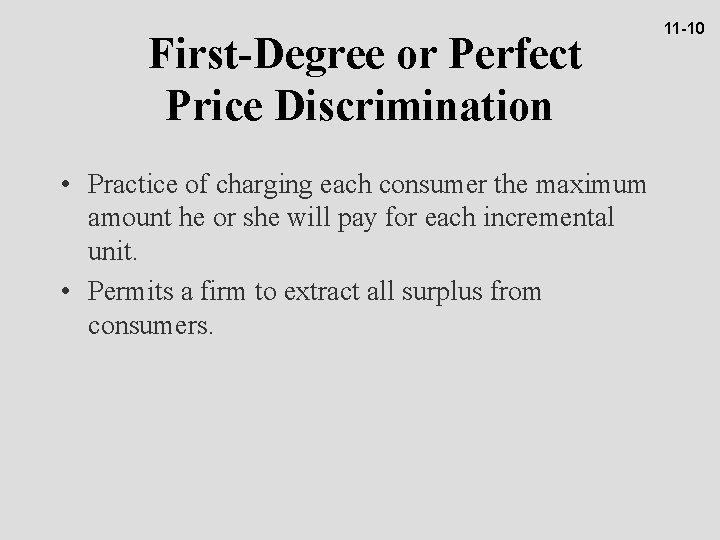First-Degree or Perfect Price Discrimination • Practice of charging each consumer the maximum amount he or she will pay for each incremental unit. • Permits a firm to extract all surplus from consumers. 11 -1011 -11 Perfect Price Discrimination Price Profits*: . 5(4 -0)(10 - 2) = \$16 10 8 6 4 Total Cost* = \$8 2 MC D 1 * Assuming no fixed costs 2 3 4 5 Quantity11 -12 Caveats: • In practice, transactions costs and information constraints make this difficult to implement perfectly (but car dealers and some professionals come close). • Price discrimination won’t work if consumers can resell the good.11 -13 Second-Degree Price Discrimination Price • The practice of posting a discrete schedule of declining prices for different quantities. • Eliminates the information constraint present in first-degree price discrimination. • Example: Electric utilities MC \$10 \$8 \$5 D 2 4 Quantity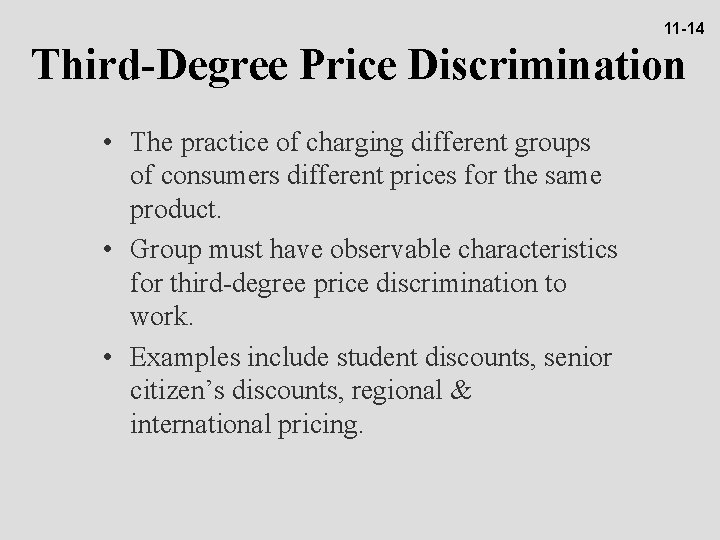11 -14 Third-Degree Price Discrimination • The practice of charging different groups of consumers different prices for the same product. • Group must have observable characteristics for third-degree price discrimination to work. • Examples include student discounts, senior citizen’s discounts, regional & international pricing.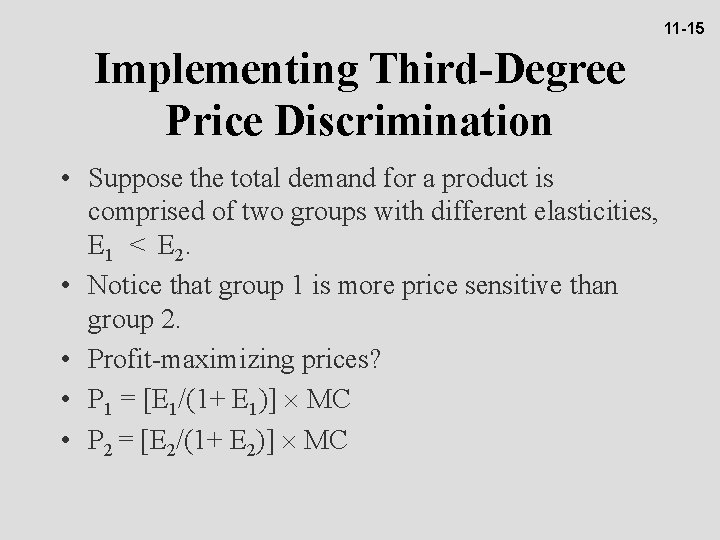11 -15 Implementing Third-Degree Price Discrimination • Suppose the total demand for a product is comprised of two groups with different elasticities, E 1 < E 2. • Notice that group 1 is more price sensitive than group 2. • Profit-maximizing prices? • P 1 = [E 1/(1+ E 1)] MC • P 2 = [E 2/(1+ E 2)] MCAn Example • Suppose the elasticity of demand for Kodak film in the US is EU = -1. 5, and the elasticity of demand in Japan is EJ = -2. 5. • Marginal cost of manufacturing film is \$3. • PU = [EU/(1+ EU)] MC = [-1. 5/(1 - 1. 5)] \$3 = \$9 • PJ = [EJ/(1+ EJ)] MC = [-2. 5/(1 - 2. 5)] \$3 = \$5 • Kodak’s optimal third-degree pricing strategy is to charge a higher price in the US, where demand is less elastic. 11 -16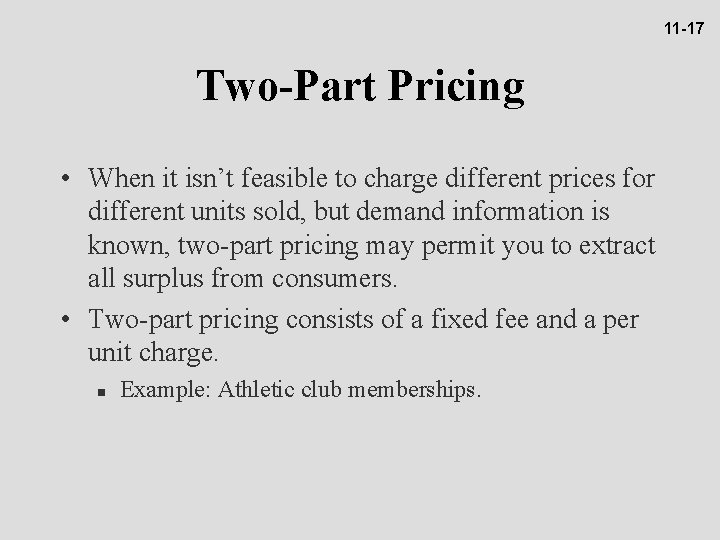11 -17 Two-Part Pricing • When it isn’t feasible to charge different prices for different units sold, but demand information is known, two-part pricing may permit you to extract all surplus from consumers. • Two-part pricing consists of a fixed fee and a per unit charge. Example: Athletic club memberships.11 -18 How Two-Part Pricing Works Price 1. Set price at marginal cost. 2. Compute consumer surplus. 3. Charge a fixed-fee equal to consumer surplus. 10 8 6 Per Unit Charge Fixed Fee = Profits* = \$16 4 MC 2 * Assuming no fixed costs D 1 2 3 4 5 Quantity11 -19 Block Pricing • The practice of packaging multiple units of an identical product together and selling them as one package. • Examples Paper. Six-packs of soda. Different sized of cans of green beans.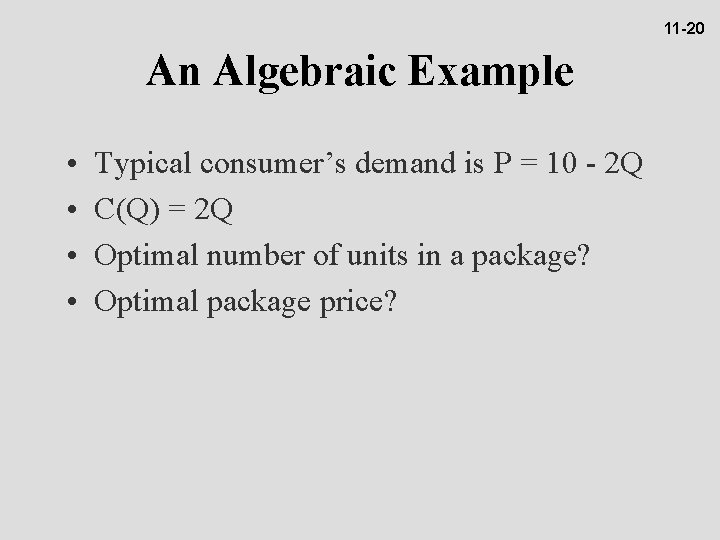11 -20 An Algebraic Example • • Typical consumer’s demand is P = 10 - 2 Q C(Q) = 2 Q Optimal number of units in a package? Optimal package price?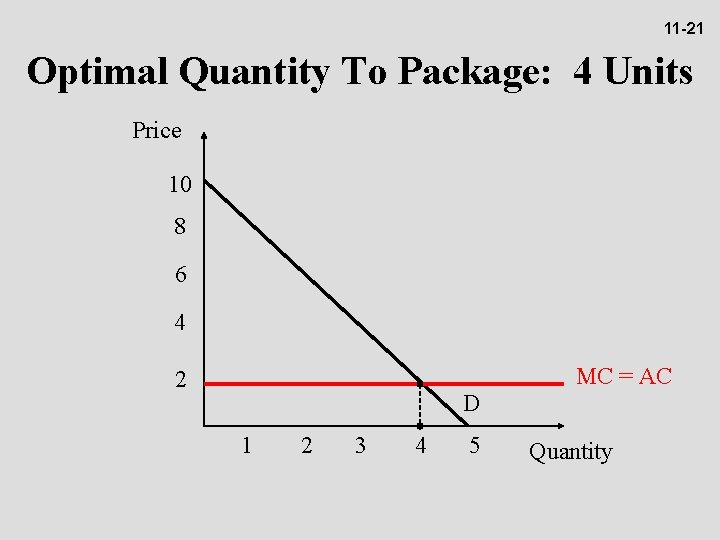11 -21 Optimal Quantity To Package: 4 Units Price 10 8 6 4 MC = AC 2 D 1 2 3 4 5 Quantity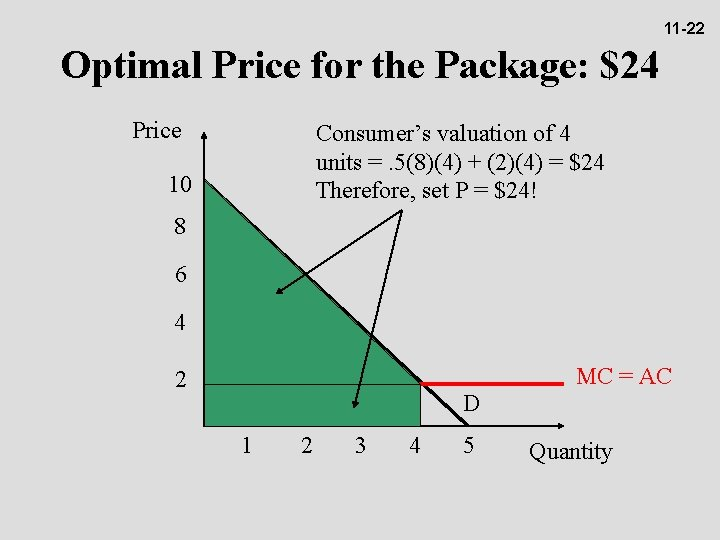11 -22 Optimal Price for the Package: \$24 Price Consumer’s valuation of 4 units =. 5(8)(4) + (2)(4) = \$24 Therefore, set P = \$24! 10 8 6 4 MC = AC 2 D 1 2 3 4 5 Quantity11 -23 Costs and Profits with Block Pricing Price 10 Profits* = [. 5(8)(4) + (2)(4)] – (2)(4) = \$16 8 6 4 Costs = (2)(4) = \$8 2 D 1 * Assuming no fixed costs 2 3 4 5 MC = AC Quantity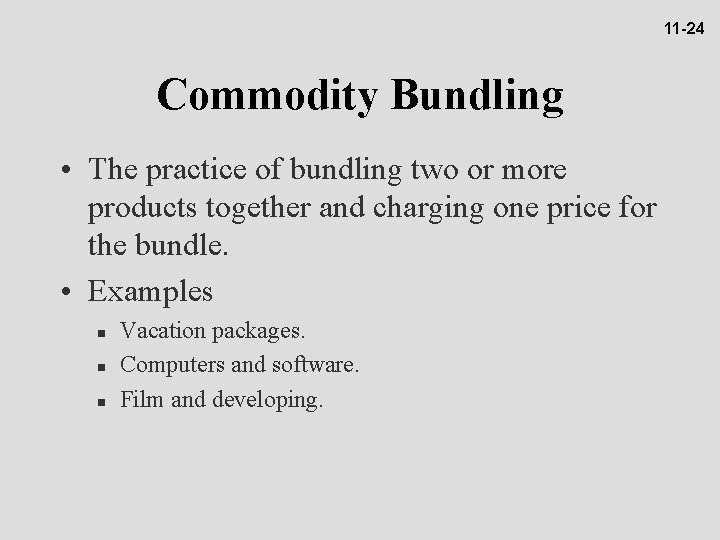11 -24 Commodity Bundling • The practice of bundling two or more products together and charging one price for the bundle. • Examples Vacation packages. Computers and software. Film and developing.An Example that Illustrates Kodak’s Moment • Total market size for film and developing is 4 million consumers. • Four types of consumers 25% will use only Kodak film (F). 25% will use only Kodak developing (D). 25% will use only Kodak film and use only Kodak developing (FD). 25% have no preference (N). • Zero costs (for simplicity). • Maximum price each type of consumer will pay is as follows: 11 -25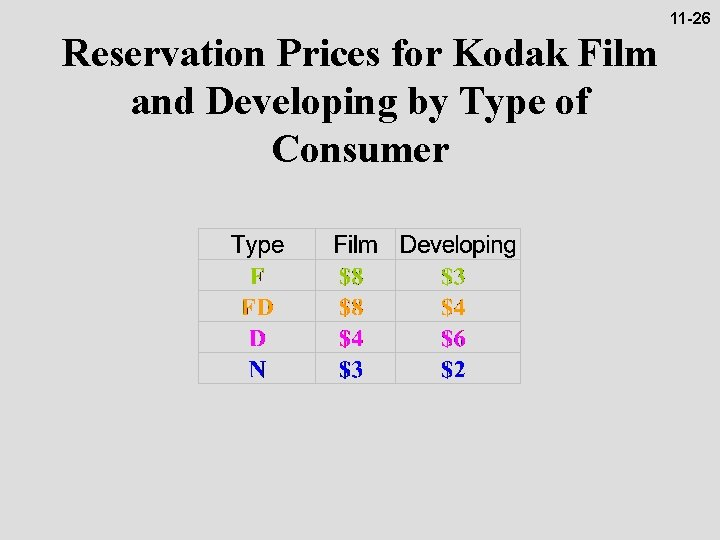11 -26 Reservation Prices for Kodak Film and Developing by Type of Consumer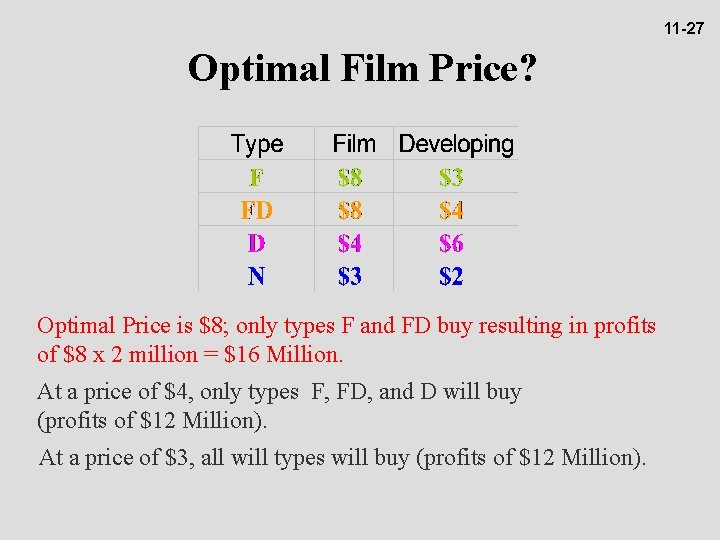11 -27 Optimal Film Price? Optimal Price is \$8; only types F and FD buy resulting in profits of \$8 x 2 million = \$16 Million. At a price of \$4, only types F, FD, and D will buy (profits of \$12 Million). At a price of \$3, all will types will buy (profits of \$12 Million).11 -28 Optimal Price for Developing? At a price of \$6, only “D” type buys (profits of \$6 Million). At a price of \$4, only “D” and “FD” types buy (profits of \$8 Million). At a price of \$2, all types buy (profits of \$8 Million). Optimal Price is \$3, to earn profits of \$3 x 3 million = \$9 Million.11 -29 Total Profits by Pricing Each Item Separately? Total Profit = Film Profits + Development Profits = \$16 Million + \$9 Million = \$25 Million Surprisingly, the firm can earn even greater profits by bundling!11 -30 Pricing a “Bundle” of Film and Developing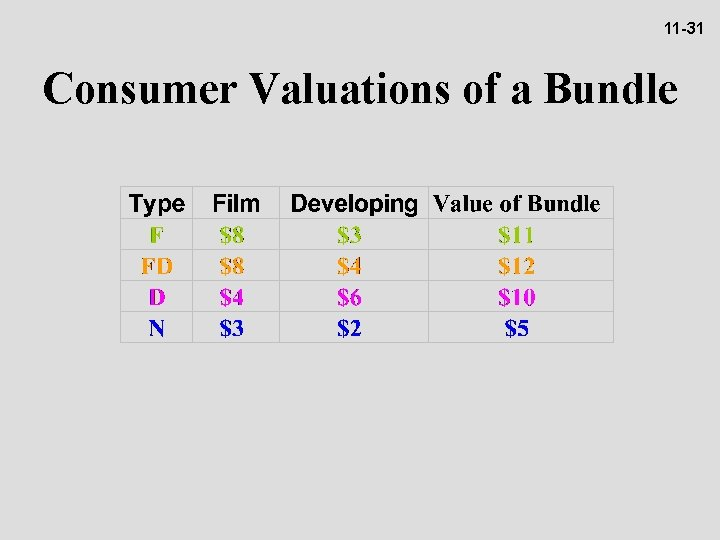11 -31 Consumer Valuations of a Bundle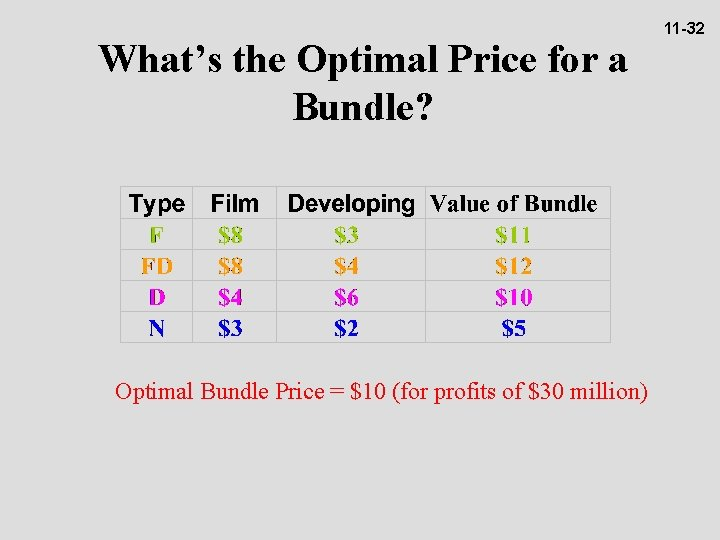What’s the Optimal Price for a Bundle? Optimal Bundle Price = \$10 (for profits of \$30 million) 11 -32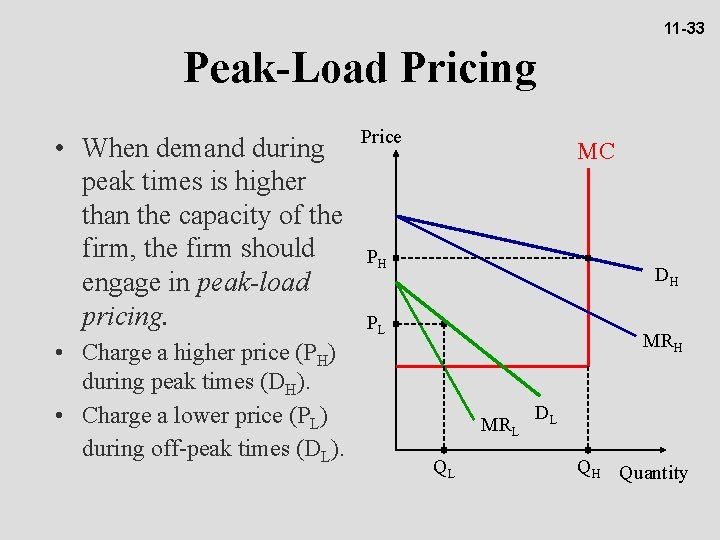11 -33 Peak-Load Pricing • When demand during peak times is higher than the capacity of the firm, the firm should engage in peak-load pricing. • Charge a higher price (PH) during peak times (DH). • Charge a lower price (PL) during off-peak times (DL). Price MC PH DH PL MRH MRL QL DL QH Quantity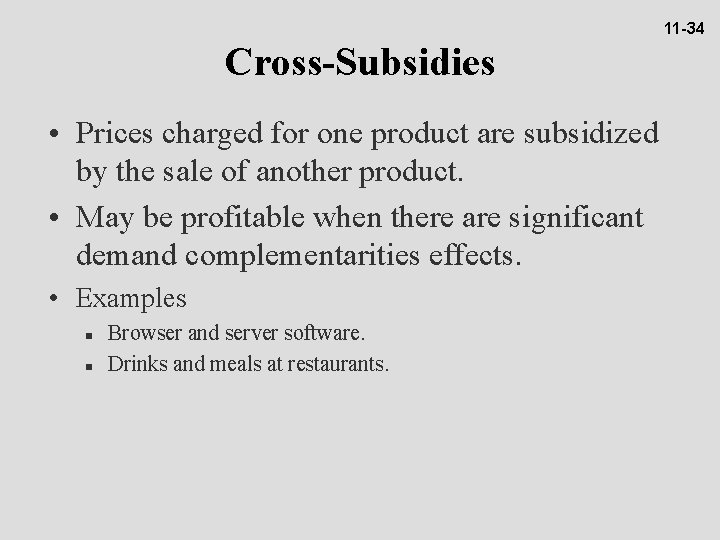11 -34 Cross-Subsidies • Prices charged for one product are subsidized by the sale of another product. • May be profitable when there are significant demand complementarities effects. • Examples Browser and server software. Drinks and meals at restaurants.11 -35 Double Marginalization • Consider a large firm with two divisions: the upstream division is the sole provider of a key input. the downstream division uses the input produced by the upstream division to produce the final output. • Incentives to maximize divisional profits leads the upstream manager to produce where MRU = MCU. Implication: PU > MCU. • Similarly, when the downstream division has market power and has an incentive to maximize divisional profits, the manager will produce where MRD = MCD. Implication: PD > MCD. • Thus, both divisions mark price up over marginal cost resulting in in a phenomenon called double marginalization. Result: less than optimal overall profits for the firm.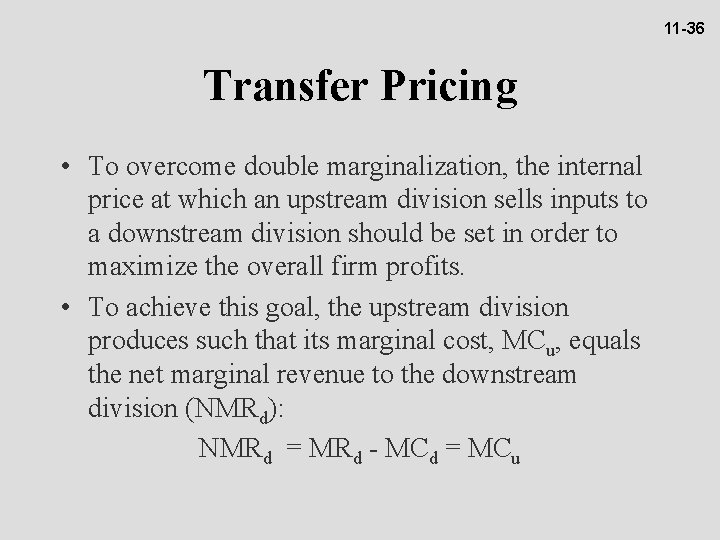11 -36 Transfer Pricing • To overcome double marginalization, the internal price at which an upstream division sells inputs to a downstream division should be set in order to maximize the overall firm profits. • To achieve this goal, the upstream division produces such that its marginal cost, MCu, equals the net marginal revenue to the downstream division (NMRd): NMRd = MRd - MCd = MCuUpstream Division’s Problem • Demand for the final product P = 10 - 2 Q. • C(Q) = 2 Q. • Suppose the upstream manager sets MR = MC to maximize profits. • 10 - 4 Q = 2, so Q* = 2. • P* = 10 - 2(2) = \$6, so upstream manager charges the downstream division \$6 per unit. 11 -37Downstream Division’s Problem • Demand for the final product P = 10 - 2 Q. • Downstream division’s marginal cost is the \$6 charged by the upstream division. • Downstream division sets MR = MC to maximize profits. • 10 - 4 Q = 6, so Q* = 1. • P* = 10 - 2(1) = \$8, so downstream division charges \$8 per unit. 11 -38Analysis • This pricing strategy by the upstream division results in less than optimal profits! • The upstream division needs the price to be \$6 and the quantity sold to be 2 units in order to maximize profits. Unfortunately, • The downstream division sets price at \$8, which is too high; only 1 unit is sold at that price. Downstream division profits are \$8 1 – 6(1) = \$2. • The upstream division’s profits are \$6 1 - 2(1) = \$4 instead of the monopoly profits of \$6 2 - 2(2) = \$8. • Overall firm profit is \$4 + \$2 = \$6. 11 -39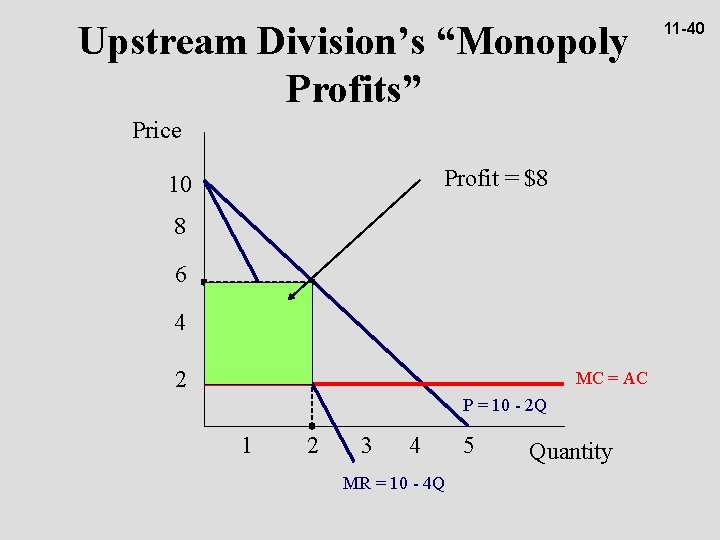Upstream Division’s “Monopoly Profits” Price Profit = \$8 10 8 6 4 2 MC = AC P = 10 - 2 Q 1 2 3 4 MR = 10 - 4 Q 5 Quantity 11 -40Upstream’s Profits when Downstream Marks Price Up to \$8 Price Downstream Price Profit = \$4 10 8 6 4 2 MC = AC P = 10 - 2 Q 1 2 3 4 MR = 10 - 4 Q 5 Quantity 11 -41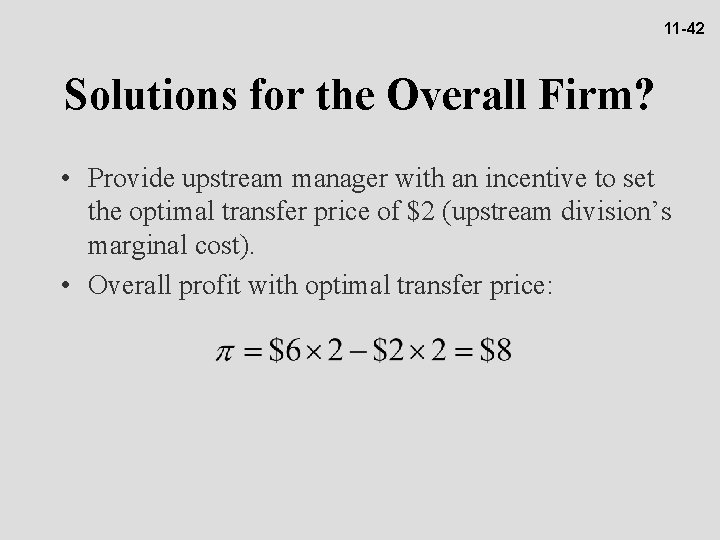11 -42 Solutions for the Overall Firm? • Provide upstream manager with an incentive to set the optimal transfer price of \$2 (upstream division’s marginal cost). • Overall profit with optimal transfer price:Pricing in Markets with Intense Price Competition 11 -43 • Price Matching Advertising a price and a promise to match any lower price offered by a competitor. No firm has an incentive to lower their prices. Each firm charges the monopoly price and shares the market. • Induce brand loyalty Some consumers will remain “loyal” to a firm; even in the face of price cuts. Advertising campaigns and “frequent-user” style programs can help firms induce loyal among consumers. • Randomized Pricing A strategy of constantly changing prices. Decreases consumers’ incentive to shop around as they cannot learn from experience which firm charges the lowest price. Reduces the ability of rival firms to undercut a firm’s prices.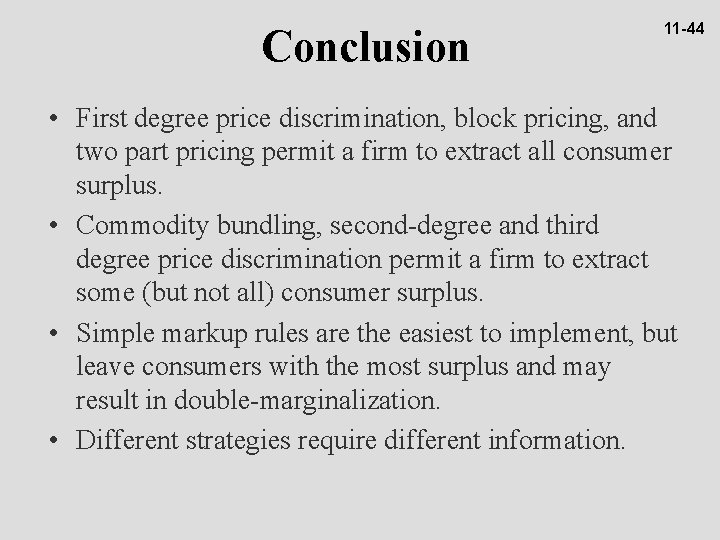Conclusion 11 -44 • First degree price discrimination, block pricing, and two part pricing permit a firm to extract all consumer surplus. • Commodity bundling, second-degree and third degree price discrimination permit a firm to extract some (but not all) consumer surplus. • Simple markup rules are the easiest to implement, but leave consumers with the most surplus and may result in double-marginalization. • Different strategies require different information.